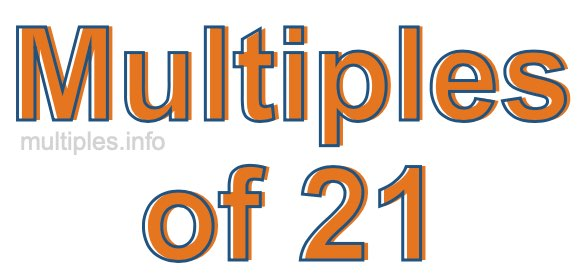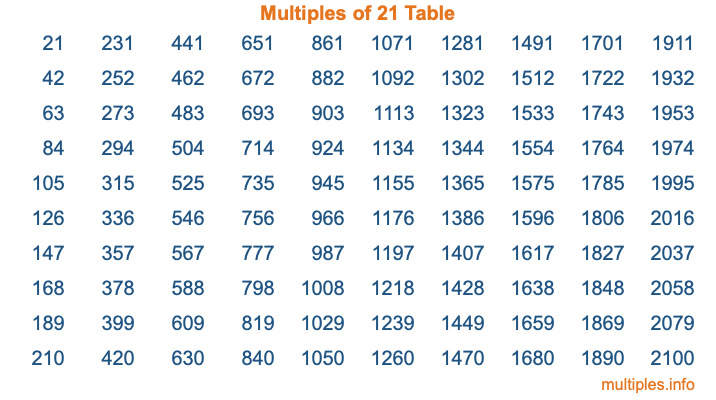Multiples of 21Welcome to the Multiples of 21 page. Here we will first teach you everything you will ever need to know about the multiples of 21, and then give you a study guide summary of everything we taught you to make sure you remember it all. Use this page to look up facts and learn information about the multiples of 21. This page will make you a multiples of twenty-one expert!

Definition of Multiples of 21
Multiples of 21 are all the numbers that when divided by 21 equal an integer. Each of the multiples of 21 are called a multiple. A multiple of 21 is created by multiplying 21 by an integer.

Therefore, to create a list of multiples of 21, you start with 1 multiplied by 21, then 2 multiplied by 21, then 3 multiplied by 21, and so on for as long as you want. Thus, the list of the first five multiples of 21 is 21, 42, 63, 84, and 105. To see a larger list of multiples of 21, see the printable image of Multiples of 21 further down on this page. We also have a category where you can choose any nth multiple of 21.

Multiples of 21 Checker
The Multiples of 21 Checker below checks to see if any number of your choice is a multiple of 21. In other words, it checks to see if there is any number (integer) that when multiplied by 21 will equal your number. To do that, we divide your number by 21. If the the quotient is an integer, then your number is a multiple of 21.

Is  a multiple of 21?

Least Common Multiple of 21 and ...
A Least Common Multiple (LCM) is the lowest multiple that two or more numbers have in common. This is also called the smallest common multiple or lowest common multiple and is useful to know when you are adding our subtracting fractions. Enter one or more numbers below (21 is already entered) to find the LCM.

Check out our LCM Calculator if you need more details about the Least Common Multiple or if you need the LCM for different numbers for adding and subtraction fractions.

nth Multiple of 21
As we stated above, 21 is the first multiple of 21, 42 is the second multiple of 21, 63 is the third multiple of 21, and so on. Enter a number below to find the nth multiple of 21.

th multiple of 21

Multiples of 21 vs Factors of 21
21 is a multiple of 21 and a factor of 21, but that is where the similarities end. All postive multiples of 21 are 21 or greater than 21. All positive factors of 21 are 21 or less than 21.

Below is the beginning list of multiples of 21 and the factors of 21 so you can compare:

Multiples of 21: 21, 42, 63, 84, 105, etc.

Factors of 21: 1, 3, 7, 21

As you can see, the multiples of 21 are all the numbers that you can divide by 21 to get a whole number. The factors of 21, on the other hand, are all the whole numbers that you can multiply by another whole number to get 21.

It's also interesting to note that if a number (x) is a factor of 21, then 21 will also be a multiple of that number (x).

Multiples of 21 vs Divisors of 21
The divisors of 21 are all the integers that 21 can be divided by evenly. Below is a list of the divisors of 21.

Divisors of 21: 1, 3, 7, 21

The interesting thing to note here is that if you take any multiple of 21 and divide it by a divisor of 21, you will see that the quotient is an integer.

Multiples of 21 Table
Below is an image of the first 100 multiples of 21 in a table. The table is in chronological order, column by column. The first column has the first ten multiples of 21, the second column has the next ten multiples of 21, and so on.The Multiples of 21 Table is also referred to as the 21 Times Table or Times Table of 21. You are welcome to print out our table for your studies.

Negative Multiples of 21
Although not often discussed or needed in math, it is worth mentioning that you can make a list of negative multiples of 21 by multiplying 21 by -1, then by -2, then by -3, and so on, to get the following list of negative multiples of 21:

-21, -42, -63, -84, -105, etc.

Multiples of 21 Summary
Below is a summary of important Multiples of 21 facts that we have discussed on this page. To retain the knowledge on this page, we recommend that you read through the summary and explain to yourself or a study partner why they hold true.

There are an infinite number of multiples of 21.

A multiple of 21 divided by 21 will equal a whole number.

21 divided by a factor of 21 equals a divisor of 21.

The nth multiple of 21 is n times 21.

The largest factor of 21 is equal to the first positive multiple of 21.

21 is a multiple of every factor of 21.

21 is a multiple of 21.

A multiple of 21 divided by a divisor of 21 equals an integer.

21 divided by a divisor of 21 equals a factor of 21.

Any integer times 21 will equal a multiple of 21.

Multiples of a Number
Here you can get the multiples of another number, all with the same attention to detail as we did for multiples of 21 on this page.

Multiples of
Multiples of 22
Did you find our page about multiples of twenty-one educational? Do you want more knowledge? Check out the multiples of the next number on our list!